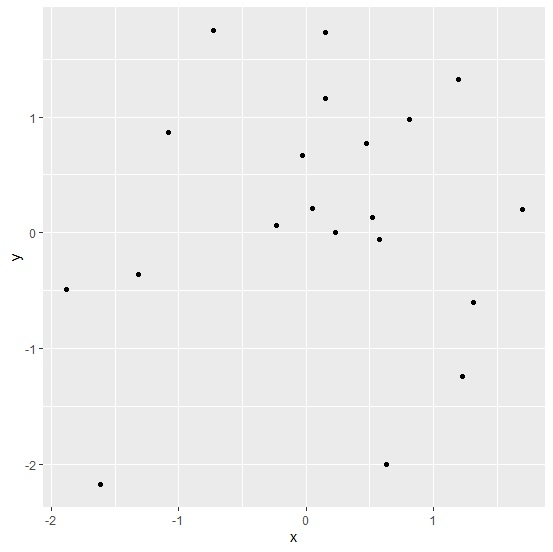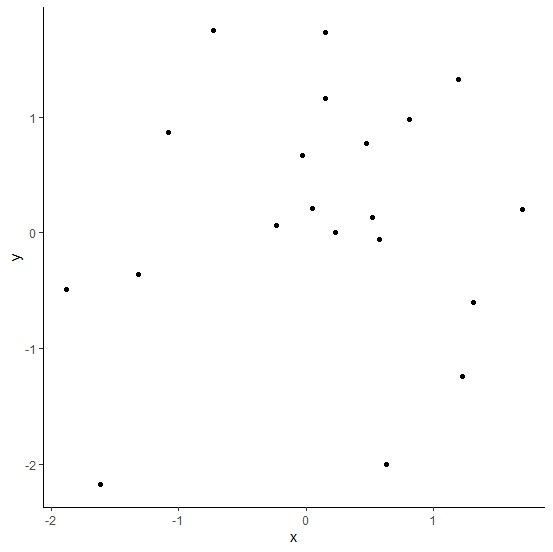# How to create a scatterplot with white background and no gridlines using ggplot2 in R?

Practically, the scatterplots are well visualized on white background just like on white paper. If we want to create a scatterplot with white background and without gridlines using ggplot2 then we can apply classic theme to the plot.

Check out the below given example to understand how it can be done.

## Example

Following snippet creates a sample data frame −

x<-rnorm(20)
y<-rnorm(20)
df<-data.frame(x,y)
df

The following dataframe is created −

        x            y
1   1.31568665  -0.606920458
2   0.15326695   1.160784530
3   0.15085999   1.729299362
4  -0.72411523   1.752459403
5   0.63320670  -2.007432892
6  -0.02838092   0.666850263
7   0.47622500   0.773645227
8  -1.31266392  -0.364087710
9  -0.23533956   0.066300263
10  0.23250476   0.002910826
11  0.05144478   0.209315785
12  1.19896603   1.322499122
13 -1.61145699  -2.178898953
14  0.57492214  -0.056533058
15 -1.88213380  -0.489842212
16  0.52139560   0.130411415
17  1.23015725  -1.240335481
18  1.70217355   0.204177146
19 -1.07961144   0.866155470
20  0.81058282   0.978253536

To load ggplot2 package and create point chart between x and y, add the following code to the above snippet −

library(ggplot2)
ggplot(df,aes(x,y))+geom_point()

## Output

If you execute all the above given snippets as a single program, it generates the following output −To create point chart between x and y with white background and no gridlines, add the following code to the above snippet −

ggplot(df,aes(x,y))+geom_point()+theme_classic()


## Output

If you execute all the above given snippets as a single program, it generates the following output −